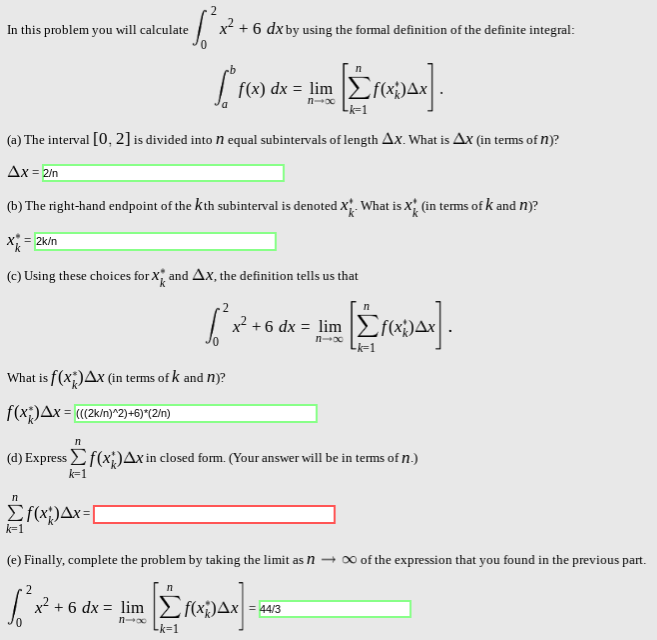# x26 dxby using the formal definition of the definite integral:In this problem you will calculateC.ebf(x) dx limn-x(a) The interval [0, 2] is divided into N equal subintervals of length Ax. What is Ax (in terms of n)?Ax = 2/n(b) The right-hand endpoint of the kth subinterval is denoted X. What is x(in tems of k and n?2k/n(c) Using these choices for Xand Ax, the definition tells us thatx2 6 dxlimn ooLi-1.f(x)Ax (in terms of k and n)?f(x)Ax = "(2k/n)^2)+6)*(2In)(d) Express(x;)Ax in closed forrm. (Your answer will be in terms of n.)k-1ITHxAx=|k 1(e) Finally, complete the problem by taking the limit as n00 of the expression that you found in the previous part.2x26 dx lim f(xAx44/3nxLk=1

Question
148 views

(d) How to express the summation: (x^(2)+6) in closed form? In terms of "n". (domain of 0 to 2)help_outlineImage Transcriptionclosex26 dxby using the formal definition of the definite integral: In this problem you will calculate C. eb f(x) dx lim n-x (a) The interval [0, 2] is divided into N equal subintervals of length Ax. What is Ax (in terms of n)? Ax = 2/n (b) The right-hand endpoint of the kth subinterval is denoted X. What is x (in tems of k and n? 2k/n (c) Using these choices for X and Ax, the definition tells us that x2 6 dx lim n oo Li-1. f(x)Ax (in terms of k and n)? f(x)Ax = "(2k/n)^2)+6)*(2In) (d) Express(x;)Ax in closed forrm. (Your answer will be in terms of n.) k-1 IT HxAx=| k 1 (e) Finally, complete the problem by taking the limit as n 00 of the expression that you found in the previous part. 2 x26 dx lim f(xAx 44/3 nx Lk=1 fullscreen
check_circle

the inegral in the close form and...

### Want to see the full answer?

See Solution

#### Want to see this answer and more?

Solutions are written by subject experts who are available 24/7. Questions are typically answered within 1 hour.*

See Solution
*Response times may vary by subject and question.
Tagged in

### Functions